5/5 - (1 vote)

Introducing the blood alcohol measurement tool, which is calculated according to the standard formula. You can use a smartphone or a computer, as long as your device has an Internet connection

## Tools to measure blood alcohol concentration

Below are some standard formulas for measuring blood levels:### #1. Formula to calculate blood alcohol concentration

C = 1,056 x A : (10W x R)

Inside:

• W:  It’s the weight.
• R:  Is the alcohol absorption constant (for men it is 0.7, for women it is 0.6)
• A: Number of alcohol units consumed. 1 unit of alcohol = 220ml of 5% beer (about 2/3 of a bottle), = 100ml of 13.5% wine or 30ml of 40% strength spirits

### #2. Formula for calculating alcohol concentration in breath

Formula: B = C : 210

### #3. The formula for calculating the elimination time of alcohol concentration in the blood

Formula: T = C : 0.015

### #4. How to use Tools to measure blood alcohol concentration

Please enter all information such as: gender, age, number of bottles of beer consumed, how long, weight…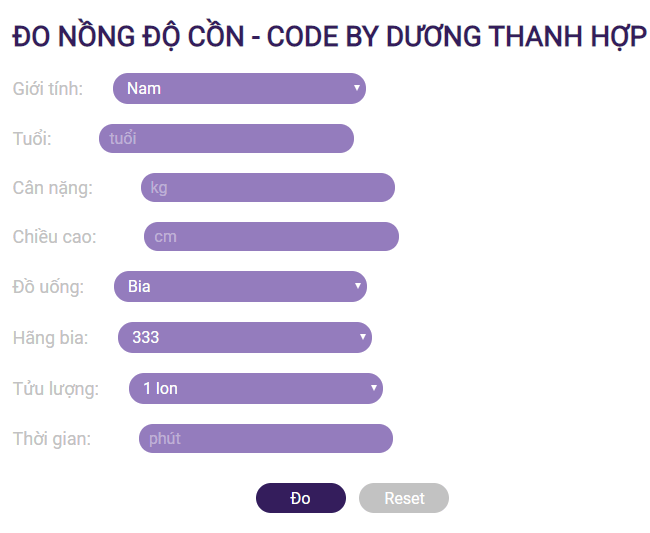Choose the brand of beer or wine you drank => After measuring, press the `ĐO` button to perform the measurement.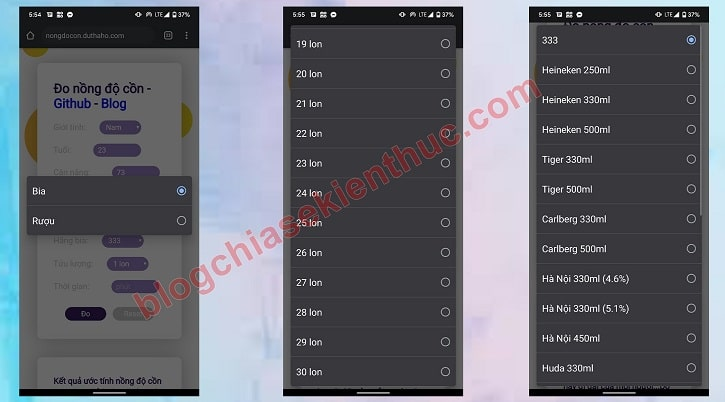Some measurement results: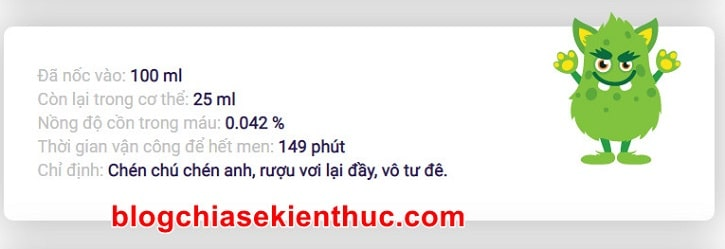Hoặc: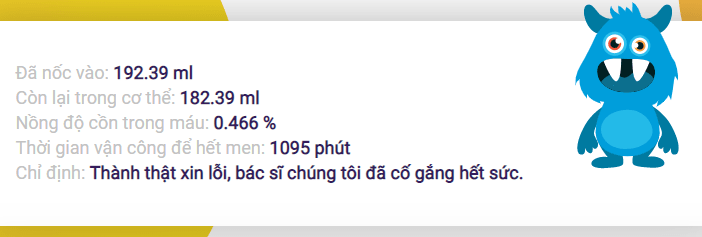Hoặc: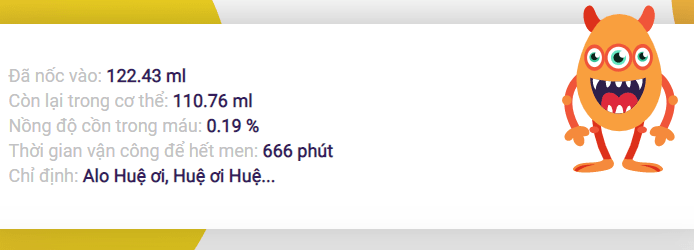Hope this online blood alcohol measurement tool will be useful to you. Thanks !